# Class 6 Maths NCERT Solutions for Chapter 14 Practical Geometry Ex – 14.2

## Practical Geometry

Question 1.
Draw a line segment of length 7.3 cm, using a ruler.

Solution:
Steps of Construction:

1. Mark a point A on the plane of the paper and place the ruler so that zero mark of the ruler is at A.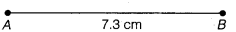1. Mark with pencil a point B against the mark on the ruler which indicates 7.3 cm.
2. Join points A and B by moving the tip of the pencil against the straight edge of the ruler.
The line segment AB so obtained is the required line segment.

Question 2.
Construct a line segment of length 5.6 cm using ruler and compasses.

Solution:
Steps of Construction:

1. Mark a point A on the plane of the paper and draw a line, say l, passing through it.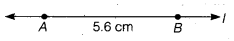2. Place the steel end of the compasses at zero mark on the ruler and open out it such that the pencil end on the mark indicates 5.6 cm.
3. Transfer the compasses as it is to the line l so that the steel end is on A.
4. With the pencil end make a small stroke on l so as to cut it at B.
5. The segment AB so obtained is the required line segment.

Question 3.
Construct$\overline { AB }$ of length 7.8 cm. From this, cut off$\overline { AC }$ of length 4.7 cm. Measure$\overline { BC }$.

Solution:
Steps of Construction:

1. Draw a line segment AB of length 7.8 cm.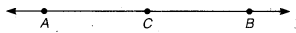2. Using compasses find a point C on the line segment AB so that segment AC = 4.7 cm.
3. On measuring BC, we find that BC = 3.1 cm.

Question 4.
Given$\overline { AB }$ of length 3.9 cm, construct$\overline { PQ }$ such that the length of$\overline { PQ }$ is twice that of$\overline { AB }$. Verify by measurement.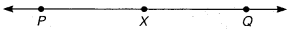Solution:
Steps of Construction:

1. Draw a line l and mark a point P on it.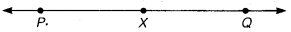2. Using compasses find a point X so that PX(= AB) = 3.9 cm on the line l.
3. Using compasses find a point Q so that XQ = 3.9 cm on the line l.
Thus, PQ = PX + XQ = 3.9 cm + 3.9 cm
= 2(3.9 cm) = 2AB.

Question 5.
Given$\overline { AB }$ of length 7.3 cm and$\overline { CD }$ of length 3.4 cm, construct a line segment$\overline { XY }$ such that the length of$\overline { XY }$ is equal to the difference between the lengths of$\overline { AB }$ and$\overline { CD }$. Verify by measurement

Solution:
Steps of Construction: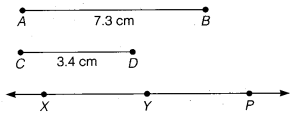1. Draw line segments AB = 7.3 cm and CD = 3.4 cm.
2. Draw a line l and mark a point X on it.
3. Using compasses find a point P on the line l so that segment XP = segment AB (i.e., 7.3 cm).
4. Using compasses find a point Y so that the segment PY = segment CD (i.e., 3.4 cm). The segment XY so obtained is the required segment, because XY = OP -PY – AB -CD.